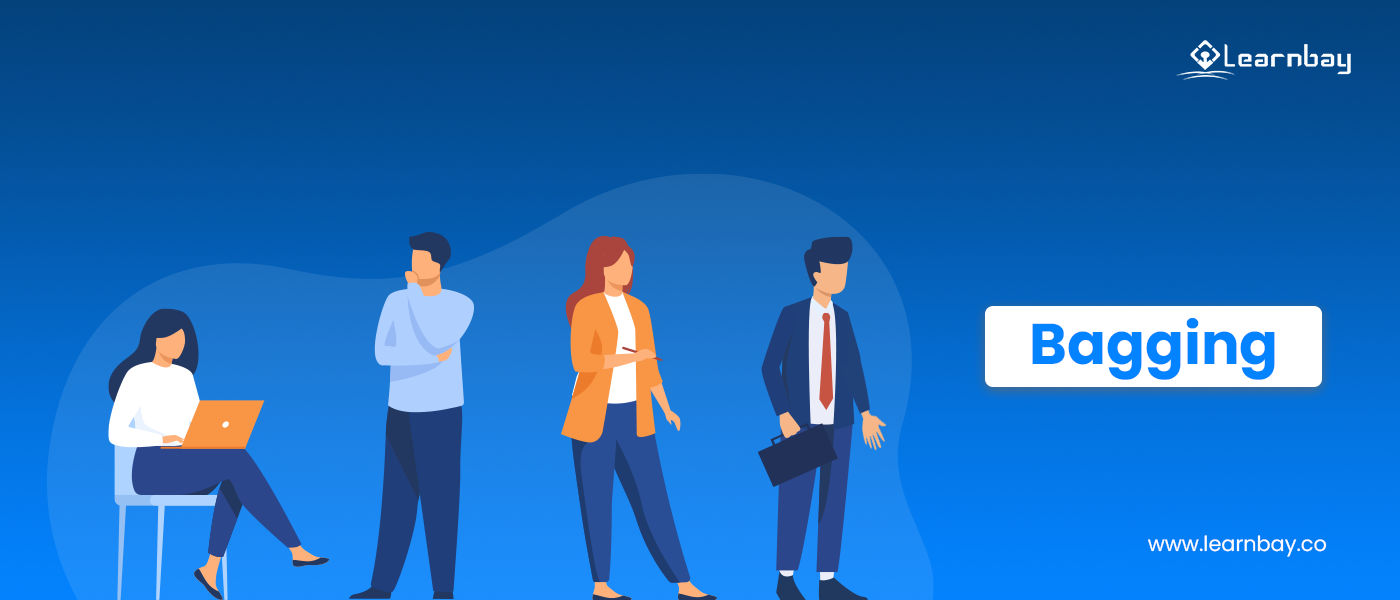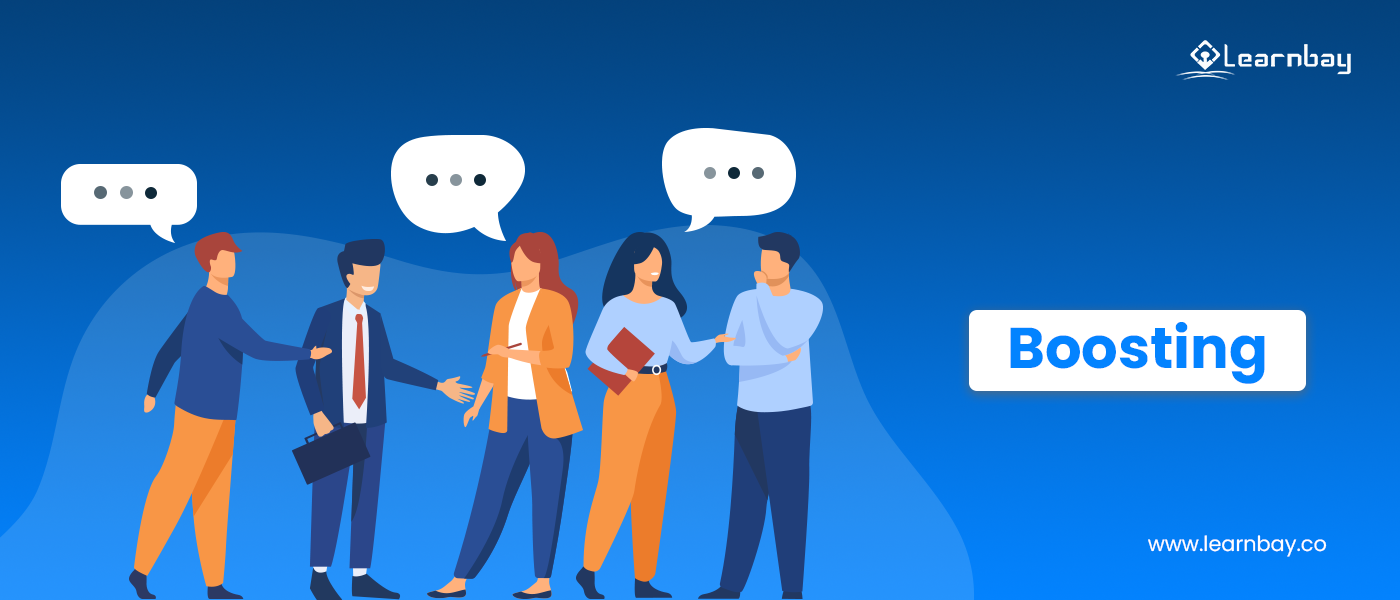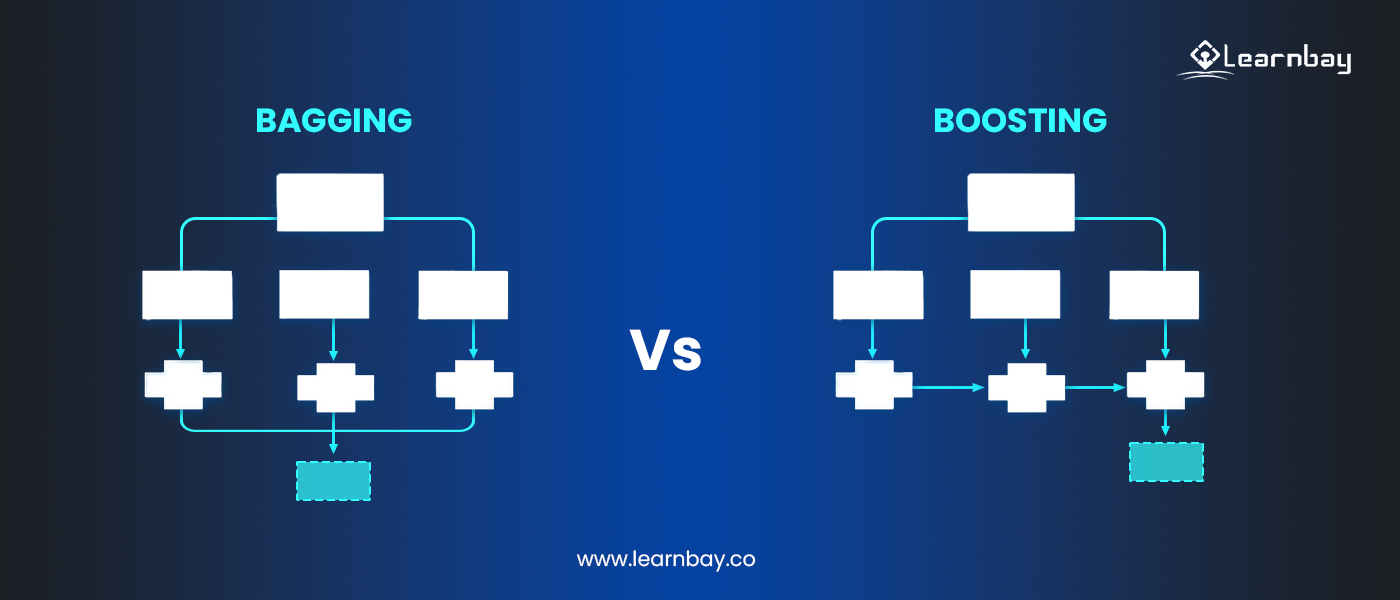# Fundamentals of Bagging and Boosting in Machine Learning | Ensemble Method

By Manas Kochar Category Machine Learning Reading time 10-12 mins Published on Mar 15, 2023

What is Bagging and Boosting in Machine Learning?

Machine learning is about enabling the systems to work without taking step-by-step instructions from a human being. This also means creating better-performing systems that produce accurate results.

If the models don't function correctly, they may produce ineffective results. The machine learning models hence are complex and require time-to-time monitoring. But some fundamentals in machine learning help us understand the model's complexity and provide solutions to the problems as well.

Here the term 'bagging and boosting' in machine learning comes into play. These are the essential components of ML that every engineer needs to understand.

They are a part of ensemble learning methods. Ensemble modeling is a technique where weak learners (or models) merge to create a strong learner.

## What is the ensemble method (learning)?

Ensemble learning is an effective approach to increasing an ML model's performance. It's nothing but merging multiple machine-learning models. Merging multiple models helps build a better prediction capability as compared to a single model.

But do you know the distinction between bagging vs. boosting in machine learning? Or what is the purpose of bagging and boosting ensemble methods?

This post will discuss all of these topics and more. But first, you need to understand these terms in detail.## What is bagging in machine learning?

Bagging (or Bootstrap Aggregation) is a simple ensemble approach, but it is extremely effective. This model learns from one another simultaneously and then combines the result to calculate the model average.

Bagging is a weak learner model. In the bagging method, we apply the bootstrap sample to a machine-learning algorithm that shows high variance. These are mainly decision trees in the case of the bagging algorithm.

It is the process of integrating the outputs of different decision trees to produce a generalized result.

## What is bootstrapping?

Bootstrapping is a simple method of sampling data into subsets and replacing this subset with a better one. This method is helpful in understanding the variance or bias present in the dataset.

A random sampling of subsets is done with the same probability as the original dataset. The subset and original set are also of the same size.

## What is boosting in machine learning?Boosting is a procedure occurring in sequential form. In this, each succeeding model will solve the preceding model's mistakes. The succeeding models rely on the preceding model.

The initial learners apply basic models to data, and the subsequent learners then examine the data to find flaws. In this way, the learners learn progressively from the other learners and produce a model with less bias at the end.

The terms bias and variance might confuse those not familiar with them. So let's discuss them in detail below.

## Variance and Bias

• When a model makes an assumption on the target function, it produces bias. This assumption helps the model learn the function easily. But if the model produces high bias, then it may not learn properly from the training data and become less flexible as a result.

• The difference in prediction results when we switch to another dataset is known as a variance. How much the model can adjust to the data for a different training set can be defined by variance. A model should generally have low variance.

Different algorithms produce different results when using the prediction algorithms for a set of data. The main point of difference between the produced results is how much bias or variance each produce.

For example, you can use either linear regression or decision trees to predict the cost of an item from a given dataset. Both these algorithms will predict different outcomes on the same dataset.

While predicting, you may observe three types of errors:

• Bias

• Variance

• Noise (fundamental error)

We can reduce bias and variance by choosing a different algorithm.

## How does the bagging method work?

1. First, we create diverse subsets from the raw dataset. We will select the observations with replacements.

2. Then, we create a base (weak) model for every subset.

3. These are independent models that run parallel to one another.

4. The predictions gained from each model combine to provide us with a final prediction with less bias.

## How does the boosting method work?

1. We create one subset from the original dataset. All the data points are provided with similar weights.

2. We create a base model that predicts the entire dataset.

3. The model calculates errors with the help of the actual and predicted values.

4. Here, those observations that show incorrect predictions are assigned higher weights.

5. Then, we create another model which will solve the issues present in the last one. It does so by making predictions on this dataset.

6. This way, we create different models that work on the errors made by the previous model.

7. We are left with a final model (strong learner). This model displays the weighted average of all the weak learners.

## Difference between bagging and boostingBagging       Boosting
In bagging, we merge predictions belonging to the same type. In boosting, we merge predictions belonging to the different types.
Bagging reduces the variance in a model. Boosting decreases the bias present in a model.
The bagging method provides each model with equal weight. The boosting method assigns weights based on the model's performance.
The models developed are not dependent on each other. The preceding models' effectiveness influences the design of the current model.
We train the base classifiers (models) parallelly. We train base models sequentially.
We use bagging when the classifier is unstable (shows high variance). We use boosting when the classifier is steady and simple (shows a high bias).
The bagging technique is useful in resolving the problem of overfitting. Boosting tries to tackle the issue of bias.

## Similarities between bagging and boosting in machine learning

Even though both the bagging and boosting methods have different uses, there are some similarities they share with each other.

1. Both of these are ensemble strategies for producing N learners from one learner.

2. Both employ random sampling to generate a large number of training datasets.

3. Both methods make the final decision by taking the average of the N learners or majority voting (selecting the majority values).

## Which method is best out of boosting and bagging?

Which approach to take depends on the circumstances present, the dataset, and their simulation. Both the bagging and boosting approach provide solutions for different case scenarios.

• If the issue is with a single model having low performance, then boosting seems to be a better option. Boosting reduces the errors in a single model and optimizes it for a combined model having fewer errors.

• If we are faced with overfitting in a model, then apply the bagging method. Boosting doesn't help solve the problem as it faces overfitting itself. Therefore, bagging is a better alternative in this scenario.

• It handles the problem of overfitting in a model.

• It can work well with higher-dimension data.

• It helps maintain accuracy for missing values.

• The classification and regression models will not have accurate values because we calculate the final prediction from the mean predictions of subgroup trees.

• It can support several loss functions.

• It's great for interactions.

• Overfitting is a risk.

• It is necessary to tune several hyper-parameters carefully.

So, to sum up, what we can say is: Bagging and boosting are both essential for ensemble learning techniques in their own ways.

Depending on your dataset, their simulation, and the circumstances provided, you may use the bagging method in the case of a random forest model, whereas for an AdaBoost model, use the boosting method.

There are many such fundamental techniques in data science and machine learning where you need to consider the appropriate method to use and get the desired outcome from a model. A thorough training in ML will help you understand the concepts with practical examples to make you industry ready.

You can check out the Advanced AI and ML course for more details on how these concepts apply to industry use cases. Enroll yourself and get the advantage of learning and practicing through experts.